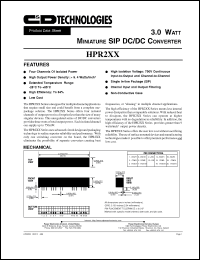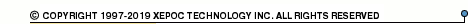More than12 555 290 queries processedHPR2 series datasheets. Manufacturer: CANDD.

 HPR203 3.0 Watt miniature DC/DC converter. Nom.input voltage 5VDC, rated output voltage +-5.2VDC, rated output current +-73mA. in 20-pin SIP package. Operational temperature range from -25°C to 85°C. Datasheet*) HPR203H 3.0 Watt miniature DC/DC converter. Nom.input voltage 5VDC, rated output voltage +-5.2VDC, rated output current +-73mA. in 20-pin SIP package. Operational temperature range from -25°C to 85°C. Datasheet*) HPR204 3.0 Watt miniature DC/DC converter. Nom.input voltage 5VDC, rated output voltage +-12VDC, rated output current +-30mA. in 20-pin SIP package. Operational temperature range from -25°C to 85°C. Datasheet*) HPR204H 3.0 Watt miniature DC/DC converter. Nom.input voltage 5VDC, rated output voltage +-12VDC, rated output current +-30mA. in 20-pin SIP package. Operational temperature range from -25°C to 85°C. Datasheet*) HPR205 3.0 Watt miniature DC/DC converter. Nom.input voltage 5VDC, rated output voltage +-15VDC, rated output current +-25mA. in 20-pin SIP package. Operational temperature range from -25°C to 85°C. Datasheet*) HPR205H 3.0 Watt miniature DC/DC converter. Nom.input voltage 5VDC, rated output voltage +-15VDC, rated output current +-25mA. in 20-pin SIP package. Operational temperature range from -25°C to 85°C. Datasheet*) HPR210 3.0 Watt miniature DC/DC converter. Nom.input voltage 12VDC, rated output voltage +-12VDC, rated output current +-30mA. in 20-pin SIP package. Operational temperature range from -25°C to 85°C. Datasheet*) HPR210H 3.0 Watt miniature DC/DC converter. Nom.input voltage 12VDC, rated output voltage +-12VDC, rated output current +-30mA. in 20-pin SIP package. Operational temperature range from -25°C to 85°C. Datasheet*) HPR211 3.0 Watt miniature DC/DC converter. Nom.input voltage 12VDC, rated output voltage +-15VDC, rated output current +-25mA. in 20-pin SIP package. Operational temperature range from -25°C to 85°C. Datasheet*) HPR211H 3.0 Watt miniature DC/DC converter. Nom.input voltage 12VDC, rated output voltage +-15VDC, rated output current +-25mA. in 20-pin SIP package. Operational temperature range from -25°C to 85°C. Datasheet*) HPR216 3.0 Watt miniature DC/DC converter. Nom.input voltage 15VDC, rated output voltage +-12VDC, rated output current +-30mA. in 20-pin SIP package. Operational temperature range from -25°C to 85°C. Datasheet*) HPR216H 3.0 Watt miniature DC/DC converter. Nom.input voltage 15VDC, rated output voltage +-12VDC, rated output current +-30mA. in 20-pin SIP package. Operational temperature range from -25°C to 85°C. Datasheet*) HPR217 3.0 Watt miniature DC/DC converter. Nom.input voltage 15VDC, rated output voltage +-15VDC, rated output current +-25mA. in 20-pin SIP package. Operational temperature range from -25°C to 85°C. Datasheet*) HPR217H 3.0 Watt miniature DC/DC converter. Nom.input voltage 15VDC, rated output voltage +-15VDC, rated output current +-25mA. in 20-pin SIP package. Operational temperature range from -25°C to 85°C. Datasheet*) HPR221 3.0 Watt miniature DC/DC converter. Nom.input voltage 24VDC, rated output voltage +-5VDC, rated output current +-75mA. in 20-pin SIP package. Operational temperature range from -25°C to 85°C. Datasheet*) HPR221H 3.0 Watt miniature DC/DC converter. Nom.input voltage 24VDC, rated output voltage +-5VDC, rated output current +-75mA. in 20-pin SIP package. Operational temperature range from -25°C to 85°C. Datasheet*) HPR223 3.0 Watt miniature DC/DC converter. Nom.input voltage 24VDC, rated output voltage +-15VDC, rated output current +-25mA. in 20-pin SIP package. Operational temperature range from -25°C to 85°C. Datasheet*) HPR223H 3.0 Watt miniature DC/DC converter. Nom.input voltage 24VDC, rated output voltage +-15VDC, rated output current +-25mA. in 20-pin SIP package. Operational temperature range from -25°C to 85°C. Datasheet*) HPR2XX 3.0 WATT MINIATURE SIP DC/DC CONVERTER Datasheet*)